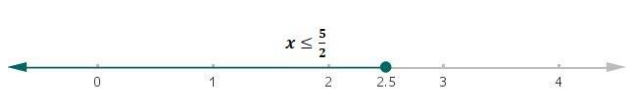# Solve each of the following in equations and represent the solution set on

Question:

Solve each of the following in equations and represent the solution set on the number line.

$\frac{5 x-8}{3} \geq \frac{4 x-7}{2}$, where $x \in \mathbf{R}$

Solution:

Given:

$\frac{5 x-8}{3} \geq \frac{4 x-7}{2}$, where $x \in R$

$(5 x-8) \cdot(2) \geq(4 x-7) \cdot(3)$

$10 x-16 \geq 12 x-21$

Now, adding 16 to both the sides

$10 x-16+16 \geq 12 x-21+16$

$10 x \geq 12 x-5$

Now, subtracting 12x from both the sides of the above equation

$10 x-12 x \geq 12 x-5-12 x$

$-2 x \geq-5$

Now, multiplying by $-1$ on both the sides of above equation

$(-2 x)(-1) \geq(-5)(-1)$

$2 x \leq 5$ (inequality reversed)

Therefore,

$x \leq \frac{5}{2}$